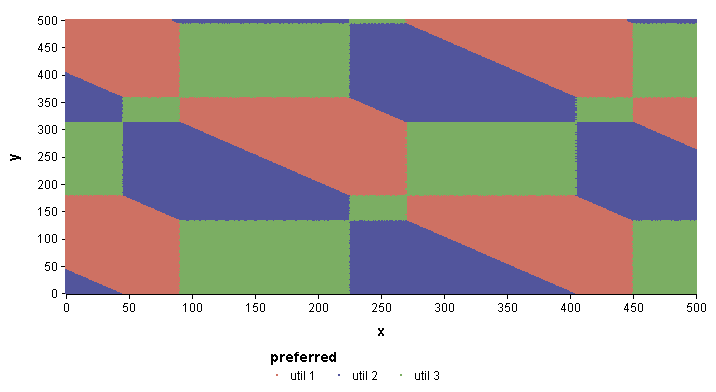# Contour/Region Charts

Contour or Region plots are not built into Analytica's graphing engine (as of Analytica 4.3). Below are two examples of these that we've "hacked" using the graphing engine in Analytica 4.2. Although not super elegant, you might come across an instance where they prove useful.A Region Plot. There are three possible options, each option being the best option in a particular region of X-Y space.A contour plot of the function Cos((X - 180)*(Y - 180)/50)

The model file for these is: Contour_region_plots.ana.

## How it is done

These were drawn by essentially plotting each pixel in the X-Y space, using the Key value to control the pixel color. X and Y are indexes that span the space of values, and the function is computed at every (X, Y) combination (every integer value in these examples).

We computed the data to plot in one variable, but I arranged the plot in a totally different node. For example, in the Region plot, the result was computed in a variable named Preferred. Its result was 2-D, indexed by X and Y, and its result table cells all contained one of three values: ['util 1', 'util 2', 'util 3'] (the color is the util curve that dominates).

To plot the region plot, I created a separate variable, named Region_plot, defined as 0 and with its domain set to be categorical. From the result view, I then pressed the XY button and added my result of interest as an external comparison variable ("Use another variable" → Add...Preferred). In Graph setup dialog, we selected Symbol graph (each pixel will be plotted using a symbol), we set "use variable symbol size" (min size = max size = 2) and "separate color/symbol keys". Then from the graph view, we pivoted so that Symbol Size Key = Region_plot, Color Key = Preferred, Horizontal Axis = X, Vertical Axis = Y, and Symbol Key = (none).

They primary trick leveraged here is the use of the Variable symbol size graphing role. This is a trick in every sense of the word since we aren't even varying the symbol size! But it is through this role that we are able to take full control of the graphing canvas in order to control each pixel individually.

Since we are creating a plot of the variable Region_plot, Analytica's graphing engine wants this value to be reflected in at least one graphing role in the plot. In most plots, it will be the vertical axis. But if you display the primary result as Symbol Size, then it will let you assign any other dimensions to the other roles, such as the two axes and the symbol color. We don't care about symbol size -- I want them all the same, so we just set the Min and Max symbol sizes to be equal (size = 2). Now, if the primary value, Region_plot, were to vary at all, then the graphing engine would also display a Key for symbol size. To eliminate the key, we just set Region_plot to have a constant value (0) and set its domain to be categorical. With only one categorical value, Analytica doesn't bother displaying a key for it. This was again a primary reason for doing this is a separate node.

The remaining configuration is all as you might expect -- nothing else really constitutes a special trick. Once you've set the symbol size as previously described, you have exactly the control over all aspects of the graph you want.

### Contour plot

The contour plot was done in exactly the same way. The only additional thing here was that the continuous values were first "discretized" into labelled bins (the z_color). Here this was done using StepInterp, which provides full control over the precise labels and ranges. You could alternatively use the Pdf or Cdf functions to automatically select the bin ranges for you. The result used for color, z_color, is actually the label for each pixel point. We've also set the domain of z_color to be an index domain, using the values from Ht. This has the effect of defining the ordering of the values in the key for z_color. (If you don't set the domain in this way, the range values will be listed in alphabetical order).